# How to Count or Sum Cells with Checked Box in Excel

This post will guide you how to count the number of checked checkboxes in your worksheet in Excel. How do I Count or sum all cells with checked boxes with a formula in Excel.

## Count or Sum Cells with Checked Box

Assuming that you have a list of data in range A1:C5, in which contain check boxes, product names and sale numbers. And you wish to count the number of all checked boxed in each row in your range. Or you want to sum all sales with checked checkboxes in your range, how to accomplish it. This post will show you one method to sum or count checked checkboxes with the following steps:

Step1: you need to select each checked box one by one, and then choose Format Control from the context menu. And the Format Control dialog will open.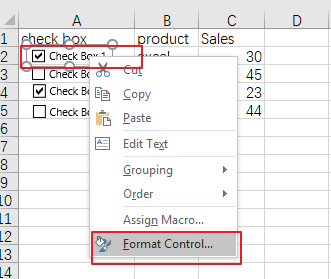Step2: click the Control tab in the Format Control dialog box, and type the cell address in the Cell link text box to link the control to a cell places the value True if the box is checked in the linked cell.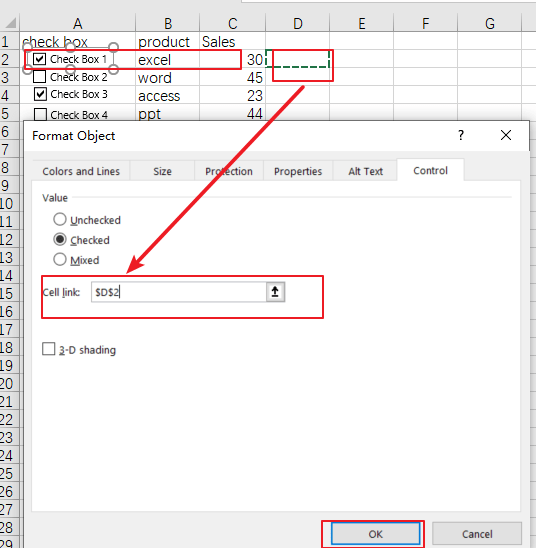Step3: you need to repeat the above two steps to set a linked cell for other checked boxes.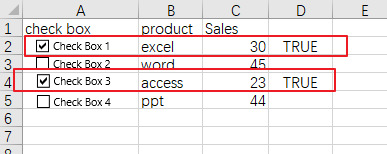Step4: then you can insert a formula based on the COUNTIF function in the blank cell that you want to place the last result, such as: Cell E1. Type the following formula into the Cell E1 to Count the number of cells with Checked box in your range A1:C5:

=COUNTIF(D2:D5,TRUE)

Then press Enter key on your keyboard to apply this formula. You would see that the number of Cells with checked checkboxes is calculated.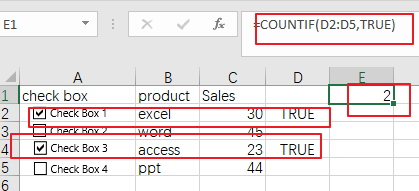Step5: if you want to sum all sales values with checked checkbox in your range, you can use another formula based on the SUMPRODUCT function to accomplish it. Like this:

=SUMPRODUCT((\$D\$2:\$D\$5=TRUE)*\$C\$2:\$C\$5)

Then you need to type this formula in the desired cell, press Enter key on your keyboard to apply it. You would see that the sum of sales value with checked checkboxes is calculated.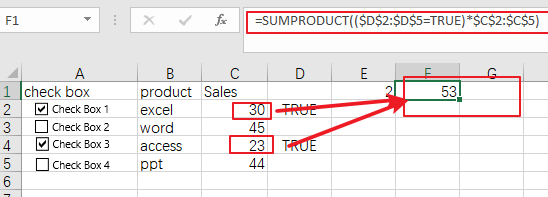Note: range D2:D5 is the range of the linked cells for checked boxes. And C2:C5 is the range of cells that contain sales values that you want to sum.

### Related Functions

• Excel SUMPRODUCT function
The Excel SUMPRODUCT function multiplies corresponding components in the given one or more arrays or ranges, and returns the sum of those products.The syntax of the SUMPRODUCT function is as below:= SUMPRODUCT (array1,[array2],…)…
• Excel COUNTIF function
The Excel COUNTIF function will count the number of cells in a range that meet a given criteria. This function can be used to count the different kinds of cells with number, date, text values, blank, non-blanks, or containing specific characters.etc.= COUNTIF (range, criteria)…

Sidebar